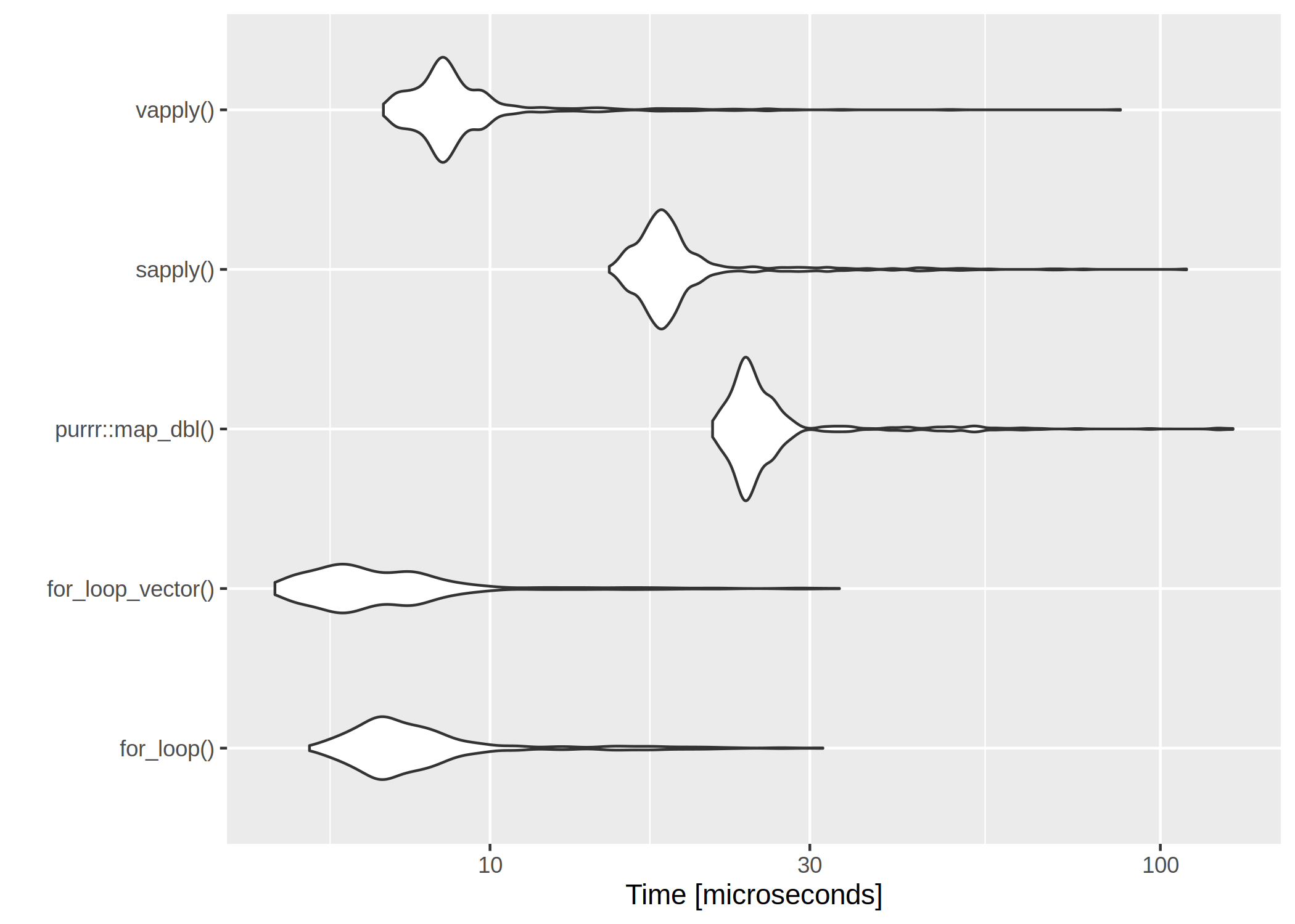# Computation time of loops — for, *apply, map

June 19, 2019
By

Want to share your content on R-bloggers? click here if you have a blog, or here if you don't.

It is usually said, that for– and while-loops should be avoided in R. I was curious about just how the different alternatives compare in terms of speed.

The first loop is perhaps the worst I can think of – the return vector is initialized without type and length so that the memory is constantly being allocated.

```use_for_loop <- function(x){
y <- c()

for(i in x){
y <- c(y, x[i] * 100)
}
return(y)
}```

The second for loop is with preallocated size of the return vector.

```use_for_loop_vector <- function(x){
y <- vector(mode = "double", length = length(x))

for(i in x){
y[i] <- x[i] * 100
}
return(y)
}```

I have noticed I use sapply() quite a lot, but I think not once have I used vapply() We will nonetheless look at both

```use_sapply <- function(x){
sapply(x, function(y){y * 100})
}

use_vapply <- function(x){
vapply(x, function(y){y * 100}, double(1L))
}```

And because I am a tidyverse-fanboy we also loop at map_dbl().

```library(purrr)
use_map_dbl <- function(x){
map_dbl(x, function(y){y * 100})
}```

We test the functions using a vector of random doubles and evaluate the runtime with microbenchmark.

```x <- c(rnorm(100))
mb_res <- microbenchmark::microbenchmark(
`for_loop()` = use_for_loop(x),
`for_loop_vector()` = use_for_loop_vector(x),
`purrr::map_dbl()` = use_map_dbl(x),
`sapply()` = use_sapply(x),
`vapply()` = use_vapply(x),
times = 500
)```

The results are listed in table and figure below.

expr min lq mean median uq max neval
for_loop() 8.440 9.7305 10.736446 10.2995 10.9840 26.976 500
for_loop_vector() 10.912 12.1355 13.468312 12.7620 13.8455 37.432 500
purrr::map_dbl() 22.558 24.3740 25.537080 25.0995 25.6850 71.550 500
sapply() 15.966 17.3490 18.483216 18.1820 18.8070 59.289 500
vapply() 6.793 8.1455 8.592576 8.5325 8.8300 26.653 500The clear winner is vapply() and for-loops are rather slow. However, if we have a very low number of iterations, even the worst for-loop isn’t too bad:

```x <- c(rnorm(10))
mb_res <- microbenchmark::microbenchmark(
`for_loop()` = use_for_loop(x),
`for_loop_vector()` = use_for_loop_vector(x),
`purrr::map_dbl()` = use_map_dbl(x),
`sapply()` = use_sapply(x),
`vapply()` = use_vapply(x),
times = 500
)```
expr min lq mean median uq max neval
for_loop() 5.992 7.1185 9.670106 7.9015 9.3275 70.955 500
for_loop_vector() 5.743 7.0160 9.398098 7.9575 9.2470 40.899 500
purrr::map_dbl() 22.020 24.1540 30.565362 25.1865 27.5780 157.452 500
sapply() 15.456 17.4010 22.507534 18.3820 20.6400 203.635 500
vapply() 6.966 8.1610 10.127994 8.6125 9.7745 66.973 500R-bloggers.com offers daily e-mail updates about R news and tutorials about learning R and many other topics. Click here if you're looking to post or find an R/data-science job.
Want to share your content on R-bloggers? click here if you have a blog, or here if you don't.